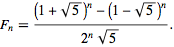# Python - How To Fibonacci Sequence in Python

ID : 368

viewed : 73

Tags : PythonPython Math95

The Fibonacci Sequence is a common and frequently used series in Mathematics. It is shown below.

``0,1,1,2,3,5,8,13,21,34,55,89,144,229.... ``

The next number in the Fibonacci Sequence is the sum of the previous two numbers and can be shown mathematically as `Fn = Fn-1 + Fn-2`.

The first and second elements of the series are 0 and 1, respectively.

In this tutorial, we will discuss how to create such a sequence in Python.

## Use the Mathematical Formula to Create a Fibonacci Sequence in Python

Every element in a Fibonacci Sequence can be represented using the following mathematical formula.We can implement this formula in Python to find the series till the required number and print the sequence. The following code shows how.

``from math import sqrt def F(n):     return ((1+sqrt(5))**n-(1-sqrt(5))**n)/(2**n*sqrt(5))  def Fibonacci(startNumber, endNumber):     n = 0     cur = F(n)     while cur <= endNumber:         if startNumber <= cur:             print(cur)         n += 1         cur = F(n) Fibonacci(1,100) ``

Output:

``1.0 1.0 2.0 3.0000000000000004 5.000000000000001 8.000000000000002 13.000000000000002 21.000000000000004 34.00000000000001 55.000000000000014 89.00000000000003 ``

The `Fibonacci()` function calculates the Fibonacci number at some position in a sequence specified by the start and end number.

## Use the `for` Loop to Create a Fibonacci Sequence in Python

We will create a function using the `for` loop to implement the required series. In this method, we will print a sequence of a required length. We will only use the `for` loop to iterate to the required length and alter the required variables every iteration. The following code explains how:

``def fibonacci_iter(n):     a=1     b=1     if n==1:         print('0')     elif n==2:         print('0','1')     else:         print('0')         print(a)         print(b)         for i in range(n-3):             total = a + b             b=a             a= total             print(total)           fibonacci_iter(8) ``

Output:

``0 1 1 2 3 5 8 13 ``

## Use a Recursive Function to Create a Fibonacci Sequence in Python

A recursive function is a function which calls itself, and such methods can reduce time complexity but use more memory. We can create such a function to return the Fibonacci Number and print the required series using a `for` loop.

For example,

``def rec_fib(n):     if n > 1:         return rec_fib(n-1) + rec_fib(n-2)     return n for i in range(10):     print(rec_fib(i)) ``

Output:

``0 1 1 2 3 5 8 13 21 34 ``

## Use Dynamic Programming Method to Create a Fibonacci Sequence in Python

Dynamic Programming is a method in which we divide problems into sub-problems and store the values of these sub-problems to find solutions. This method is usually used in optimizing problems and can be used to generate the Fibonacci Sequence as shown below:

``def fibonacci(num):     arr = [0,1]     if num==1:         print('0')     elif num==2:         print('[0,','1]')     else:         while(len(arr)<num):             arr.append(0)         if(num==0 or num==1):             return 1         else:             arr=0             arr=1             for i in range(2,num):                 arr[i]=arr[i-1]+arr[i-2]             print(arr)  fibonacci(10) ``

Output:

``[0, 1, 1, 2, 3, 5, 8, 13, 21, 34] ``

Note that the sequence is stored in an array in this method.